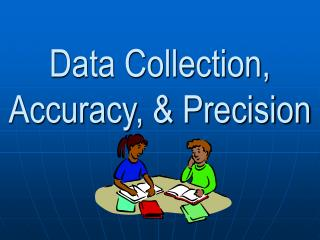Download PresentationData Collection, Accuracy, & PrecisionData Collection, Accuracy, & Precision - PowerPoint PPT Presentation

Download PresentationData Collection, Accuracy, & Precision
An Image/Link below is provided (as is) to download presentation

Download Policy: Content on the Website is provided to you AS IS for your information and personal use and may not be sold / licensed / shared on other websites without getting consent from its author. While downloading, if for some reason you are not able to download a presentation, the publisher may have deleted the file from their server.

- - - - - - - - - - - - - - - - - - - - - - - - - - - E N D - - - - - - - - - - - - - - - - - - - - - - - - - - -
Presentation Transcript

1. Data Collection, Accuracy, & Precision

2. Data Collection • There is no such thing as a perfect measurement. Each measurement contains a degree of uncertainty due to the limits of instruments and the people using them. • When an experiment is performed, it is necessary to properly collect data so that a correct conclusion can be determined.

3. Aspects of Proper Data Collection • Things to be considered in collecting data: • Using the proper SI units • Example: centimeters, not inches • Recording units with each measurement • Always include a UNIT!!! • Using the appropriate instrument • Example: graduated cylinder, not beaker

4. Aspects of Proper Data Collection (continued) • Accurately reading the instrument • Example: knowing what each line on a metric ruler represents • Writing down the known digits of the measurement plus the estimated digit • Example: making a length measurement, and estimating the last digit as either 0 or 5

5. Accuracy & Precision • Accuracy is how close a measurement, or experimental value, is to an accepted value, or standard. • Precision is how close a set of measurements are to one another.

6. Seeing Accuracy & Precision

7. Determining Trends in Data

8. Trends • When analyzing a graph, the ways that the data sets (dependent variable and independent variable) relate to one another are called trends • Trends can also be seen as “patterns” in your data • Types of trends • Positive correlation (direct, exponential) • Negative correlation (inverse, indirect or exponential)

9. Positive Correlation • When the dependent variable increases as the independent variable increases • If the variables increase at the same rate, it is a direct relationship

10. Negative Correlation • When the dependent variable decreases as the independent variable increases (this example shows an indirect relationship)

11. Stating the Trend • Instead of simply saying if a trend is positive or negative, we can put the trend into a sentence using the format below: • As the “independent variable” increases/decreases the “dependent variable” increases/decreases. (choose one) (choose one)

12. Practice • (independent variable) (circle one) • (dependent variable) (circle one) As the _____________ increases/decreases the _____________ increases/decreases. WRITE YOUR OWN TREND STATEMENT

13. Practice Based on your two trend statements, do you see a connection between the amount of rainfall and the number of Mosquito incidents?

14. What if there isn’t a distinct trend? • If you find that a trend is not clear with your graph, you should assess it based on where the pattern lies • Look at the common/frequent direction of the graph • Try to fit a line that will go in between the points… • And assess from there